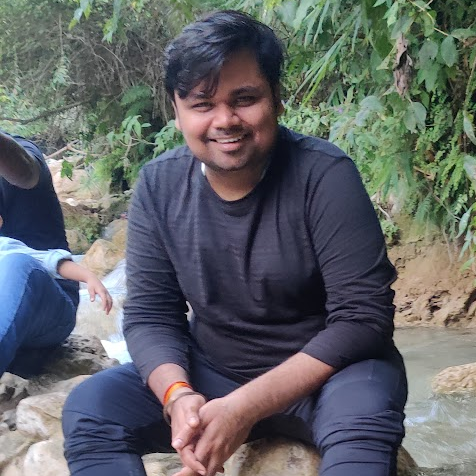SAURABH KUMAR

Let two numbers are a and b

so A/C to Qn

a^2 + b^2 = 164.......(i)

and LCM x HCF = a x b, So, a x b = 2x40.......(ii)

so, equation (i) can be written as

(a+b)^2-2ab = 164

=>(a+b)^2-2(2x40) = 164 from (ii)

=>(a+b)^2 = 164 + 160 =>324

=>(a+b) = 18.........(iii)

from (ii) and (iii)

a = 8

b = 10

Sep 23, 2018 14:47

To find the greatest common divisor, we will use the concept of H.C.F.

Let us assume R is the remainder in each case and N is the divisor.

So, (125-R), (218-R), (280-R) and (342-R) will have common factors.

when two different numbers, say X, Y are divisible by N, then their difference is also divisible by N.

so,

(218-R) - (125-R) = 93

(280-R) – (218-R) = 62

(342-R) – (280-R) = 62

Hence, N is the H.C.F of 93 and 62

93 = 3 x 31

62 = 2 x 31

Therefore, 31 is the greatest number that divides the four given numbers and leaves the same reminder i.e. 1
Sep 20, 2018 19:32

A+4=F
I+4=N
E+4=J
Similarly
K+4=P
S+4=X
0+4=T

PXT (Ans)

Sep 20, 2018 19:26

amount remaining= 24000-24000/3=16000
1. 16000/2=8000 SI=PRT/100=8000*15*1/100=1200
2. 8000/2=4000 SI=4000*10*1/100=400
3. 4000 SI=4000*4*1/100=160
SI=PRT/100 1760=16000*R*1/100
Solving this R=11%
Sep 20, 2018 19:24

Let cost of each shirt is 100
60*100=50*x ==> x=120(new cost)
20% is the profit. i.e., 20/100 = 0.2
Sep 20, 2018 19:20

E=F

X=Y

A=B

M=N

Similarly

T=U

E=F

S=T

T=U

Sep 20, 2018 19:16

Profit of 20% means he should sell the 12 clips for 72 Rs

so cost of one clips should br 72/12 i.e 6.
so for 60 Rs he should sell 10 clips

Sep 20, 2018 19:11

Here, C is coded as G which is in fourth position after C, O is coded as K which is in 5th position before O.

Following the same sequence, SENATE is coded as WARXWXA.

In this, W is 4th position after S, A is in fifth position before E and so on.
Sep 20, 2018 19:03

as 51=sum of run/matches. (1)
54= (sum+78)/matches+1 (2)
on solving the 2 equations we get matches=8
Sep 20, 2018 18:56

moving opposite direction so,
18t+20t=95
t=95/38
t=2.5 min
Sep 20, 2018 18:53

S.P=(8000*10/100)-1200
=7200-1200
=6000.
C.P=(100/100+PROFIT PERCENTAGE)*S.P
=(100/120)*6000
=5000.
Sep 20, 2018 18:50

The part of tank filled by Pipe A in 1 min = 1/20
The part of tank filled by Pipe B in 1 min = 1/30
The part of tank emptied by Pipe C in 1 min = 1/15
So totally in 3 minute (because open for 1 minute each)=1/20+1/30-1/15=1/60.
so consider 55/60 can be filled in 3*55=165min,remaining part is 1/12.
A can fill in 1 min is 1/20,so in 1min A can fill (1/12-1/20) so finally left is 1/30 so B can fill remaining 1/30 in 1 Minute
So total time taken=(3*55+1+1)=167min. So within 167min it can fill the tank.
Sep 20, 2018 18:22

722-282=50x

440=50x

x=440/50

x=8.8

Sep 20, 2018 18:16

No of odd digits between 0 to 9 is= 5 (1,3,5,7,9)
Favorable case of selecting 2 odd digits is p(A) = 5C2 = 10

and sample case of arranging two odd digits is p(S) = 5*4 = 20

So,
Probability p(N) = p(A) / p(S) = 10 / 20 = 1/2 Answer
Sep 20, 2018 18:10

4c1*5c1=20 (combination)
Sep 20, 2018 18:04

total no of ways is 8!.
for finding even nos at last 4 digit s ways are 4!
and that for odd is 4!
so it can be done in 4*3*2*1*4*3*2*1/8*7*6*5*4*3*2*1
ie 4!*4!/8! =576/40320

Sep 20, 2018 18:02

since they are directly proportional
6/21=x/14
x=(14*6)/21=4
Sep 20, 2018 17:52

compare P3M with R3P then N4J=P4M
Sep 20, 2018 17:38

5 per 0.5 kg so
total =(4.2/0.5)*0.5 =42
Sep 20, 2018 17:29

S+1 -> T
K+1 -> L
on Cross
S -> L
K -> T
similarly
SK IL LF UL
TL JM MG VM
on Cross
LT MJ GM MV
Similarly
ST AT ED
TU BU FE
on Cross
UTUBEF = Ans
Sep 16, 2018 11:35

If CP of 1 bedsheet=x,

then CP of 15 bedsheet=15x
15000-5x=15x,

20x=15000,

Sep 16, 2018 11:32

According to the given condition
a^2+b^2+c^2=170
ab+bc+ca=157
now we know that (a+b+c)^2 = a^2+b^2+c^2+2(ab+bc+ca)
on taking values we get (a+b+c)^2 = 170+2(157) = 484
or a+b+c = 22
Sep 16, 2018 11:29

sp=2400
profit=33.33%=(100+33.33)=133.33
cp=(100*2400)/133.33
=1800.04 (cp for 2t-shirt)
so cp for 1t-shirt= 900
Sep 16, 2018 11:21

Ayodhya (About this sound listen (help·info); IAST Ayodhyā) is a city located near Faizabad in Uttar Pradesh, India. It is identified with the legendary city of Ayodhya, and as such, is believed to be the birthplace of Rama and setting of the epic Ramayana.
Aug 11, 2018 10:50

KYA BEKAR QUESTION H BEWKOOF NE SESSION HI NHI MENTION KRA??? KB KA CM SELECT KRU????????
Jan 15, 2018 08:38